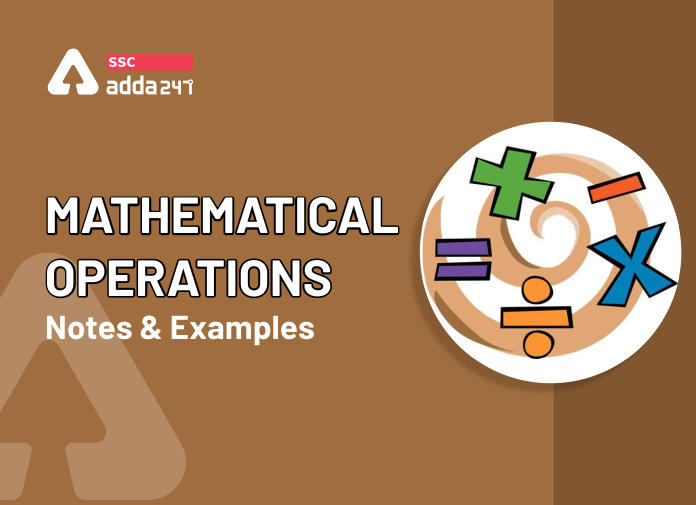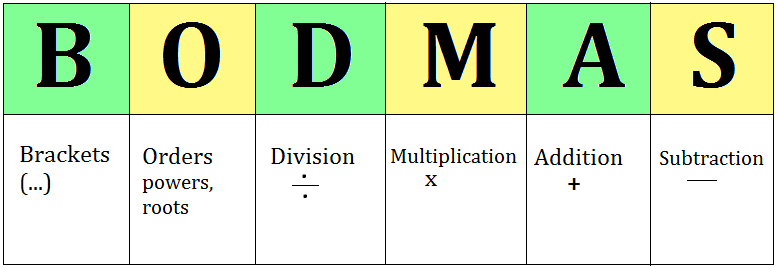Latest SSC jobs   »   Reasoning Questions Quiz for SSC CGL,...   »   Mathematical Operations

# Mathematical Operations: Notes And Examples 2021## Mathematical Operations

Mathematical Operation is an important concept of reasoning that is usually asked in various competitive exams. This topic is asked to test the analytical abilities of the candidates. It shows how good you are at observing things and then implying it to solve the questions. To score full marks on this topic, you must practice enough questions and get acquainted with the concept behind it. Here we are providing you with the method to solve mathematical operations questions along with examples.

### How to solve questions based on Mathematical Operations?

The type of questions based on Mathematical operations are:

1. Whether the given equations are correct
2. Based on Symbols equivalent to signs
3. Interchanging the signs
4. Balancing the equation
5. Solve the equation

For every type of Mathematical operations question, you must know only one rule i.e. BODMAS. It is “Brackets, Orders, Division, Multiplication, Addition, and Subtraction. It means you must solve any equation in the BODMAS order. First, open the brackets, then solve the powers or roots, then perform Division followed by multiplication, Addition and subtraction.Q1. If × stands for -, ÷ stands for +, + stands for ÷ and – stands for ×, which one of the following equations is correct?
(a) 15 – 5 ÷ 5 × 20 + 16 = 6
(b) 8 ÷ 10 – 3 + 5 × 6 = 8
(c) 6 × 2 + 3 ÷ 12 – 3 = 15
(d) 3 ÷ 7 – 5 × 10 + 3 = 10
Ans.(b)
Sol. Using the proper signs, we get:
Expression in (a) = 15 × 5 + 5 – 20 ÷ 10 = 15 × 5 + 5 – 2 = 75 + 5 – 2 = 78
Expression in (b) = 8 + 10 × 3 ÷ 5 – 6 = 8 + 10 × 3/5 – 6 = 8 + 6 – 6 = 8
Expression in (c) = 6 – 2 ÷ 3 + 12 × 3 = 6 – 2/3 + 36 = 42 – 2/3=124/3
Expression in (d) = 3 + 7 × 5 – 10 ÷ 3 = 3 + 7 × 5 – 10/3=3+35-10/3=104/3
∴ Statement (b) is true.Q2. If ‘<’ means ‘minus’, ‘>’ means ‘plus’, ‘=’ means ‘multiplied by’ and ‘\$’ means ‘divided by’, then what would be the value of 31 > 81 \$ 9 < 7?
(a) 32
(b) 33
(c) 36
(d) None of these
Ans.(b)
Sol. Using the correct symbols we have:
Given expression = 31 + 81 ÷ 9 – 7 = 31 + 9 – 7 = 33Q3. If × means ÷, – means ×, ÷ means + and + means -, then (4 – 15 ÷ 12) × 8 + 9 = ?
(a) -1
(b) 2
(c) 0
(d) 1
Ans.(c)
Sol. Using the correct symbols, we have:
Given expression = (4 × 15 + 12) ÷ 8 – 9 = 72 ÷ 8 – 9 = 9 – 9 = 0

Q4. If Q means ‘add to’, J means ‘multiply by’, T means ‘subtract from’ and K means ‘divide by’, then 26 K 2 Q 3 J 6 T 4 = ?
(a) 10
(b) 28
(c) 30
(d) 27

Ans.(d)
Sol. Using the correct symbols, we have:
Given expression = 26 ÷ 2 + 3 × 6 – 4 = 13 + 18 – 4 = 27

Q5. If ‘-’ stands for ‘division’, ‘+’ for ‘multiplication’, ‘÷’ for ‘subtraction’ and ‘×’ for ‘addition’, which one of the following equations is correct?
(a) 6 + 20 – 12 ÷ 7 – 1 = 38
(b) 6 – 20 ÷ 12 × 7 + 1 = 57
(c) 6 + 20 – 12 ÷ 7 × 1 = 62
(d) 6 ÷ 20 × 12 + 7 – 1 = 70

Ans.(d)
Sol. Using the proper notations in (d), we get the statement as:
6 – 20 + 12 × 7 ÷ 1 = 6 – 20 + 84 = 90 – 20 = 70

Q6. If L denotes ÷, M denotes ×, P denotes + and Q denotes -, then which of the following statements is true?
(a) 32 P 8 L 16 Q 4 =-2/3
(b) 6 M 18 Q 26 L 13 P 7 =173/13
(c) 11 M 34 L 17 Q 8 L 3 =38/3
(d) 9 P 9 L 9 Q 9 M 9 = -71

Ans.(d)
Sol. Using the proper notations in (d), we get the statement as:
9 + 9 ÷ 9 – 9 × 9 = 9 + 1 – 9 × 9 = 9 + 1 – 81 = 10 – 81 = -71.

Q7. Which one of the four interchanges in signs and numbers would make the given equation correct?
3 + 5 – 2 = 4
(a) + and –, 2 and 3
(b) + and –, 2 and 5
(c) + and –, 3 and 5
(d) None of these

Ans.(c)
Sol. By making the interchanges given in (a)
2 – 5 + 3 = 4 or 0 = 4, which is false.
By making the interchanges given in (b)
3 – 2 + 5 = 4 or 6 = 4, which is false.
By making the interchanges given in (c)
5 – 3 + 2 = 4 or 4 = 4, which is true.

Directions (8): In this question, ∆ means ‘is greater than’, % means ‘is lesser than’, ⃞ means ‘is equal to’, = means ‘is not equal to’, + means ‘is a little more than’, × means ‘is a little less than’. Choose the correct alternative.

Q8. If a ∆ b and b + c, then
(a) a % c
(b) c + a
(c) c % a
(d) Can’t say

Ans.(c)
Sol. a ∆ b -> a > b and
b + c -> b is a little more than c
⇒ a > c ⇒ c < a i.e. c % a

Directions (9-10): In each of the following questions, the Greek letters standing for arithmetical operations are given. Find the relationship which can definitely be deduced from the two relationships given at the top.

Operations: α is ‘greater than’, β is ‘less than’, γ is ‘not greater than’, δ is ‘not less than’, θ is ‘equal to’.
Q9. If A α 2C and 2A θ 3B, then
(a) C β B
(b) C δ B
(c) C α B
(d) C θ B

Ans.(a)
Sol. A α 2C ⇒  A > 2C
and 2A θ 3B ⇒  2A = 3B
⇒ 2A > 4C and 2A = 3B
⇒ 3B > 4C ⇒ C < B i.e. C β B

Q10. If B θ 2C and 3C γ A, then
(a) B δ 2A
(b) B θ A
(c) 3B α 2A
(d) B β A

Ans.(d)
Sol. B θ 2C⇒ B = 2C
and 3C γ A ⇒ 3C ⊁ A
⇒B = 2C and 3C ≤ A
⇒ B = 2C < 3C ≤ A ⇒ B < A i.e. B β A

Directions (11): In this question, α stands for ‘equal to’; β for ‘greater than’; γ for ‘less than’ and δ for ‘not equal to’.

Q11. If 6x α 5y and 2y β 3z, then
(a) 2x β 3z
(b) 4x β 3z
(c) 2x γ z
(d) 4x α 3z

Ans.(b)
Sol. 6x α 5y ⇒ 6x = 5y
and 2y β 3z ⇒ 2y > 3z
⇒ 6x = 5y and y>3z/2
⇒ 6x = 5y and 5y >15z/2⇒6x>15z/2
⇒ 12x > 15z ⇒ 4x > 5z
⇒ 4x > 3z i.e. 4x β 3z

Directions (12): In this question, if the given interchanges are made in signs and numbers, which one of the four equations would be correct?

Q12. Given interchanges : Signs + and – and number 4 and 8
(a) 4 ÷ 8 – 12 = 16
(b) 4 – 8 + 12 = 0
(c) 8 ÷ 4 – 12 = 24
(d) 8 – 4 ÷ 12 = 8

Ans.(b)
Sol. On interchanging + and – and 4 and 8 in (b), we get the equation as:
8 + 4 – 12 = 0 or 12 – 12 = 0 or 0 = 0, which is true

Q13. If ÷ implies =, × implies <, + implies >, – implies ×, > implies ÷, < implies +, = implies -, identify the correct expression.
(a) 1 – 3 > 2 + 1 – 5 = 3 – 1 < 2
(b) 1 – 3 > 2 + 1 × 5 = 3 × 1 > 2
(c) 1 × 3 > 2 + 1 × 5 × 3 – 1 > 2
(d) 1 – 3 > 2 + 1 × 5 + 3 – 1 > 2

Ans.(d)
Sol. Using the proper notations in (d), we get the statement as:
1 × 3 ÷ 2 > 1 < 5 > 3 × 1 ÷ 2 or 3/2>1<5>3/2, which is true.

Q14. If > denotes +, < denotes -, + denotes ÷, – denotes =, = denotes ‘less than’ and × denotes ‘greater than’, find which of the following is a correct statement.
(a) 3 + 2 > 4 = 9 + 3 < 2
(b) 3 > 2 > 4 = 18 + 3 < 1
(c) 3 > 2 < 4 × 8 + 4 < 2
(d) 3 + 2 < 4 × 9 + 3 < 3

Ans.(c)
Sol. Using proper notations, we have:
(a) Given statement is 3 ÷ 2 + 4 < 9 ÷ 3 – 2 or 11/2 < 1, which is not true
(b) Given statement is 3 + 2 + 4 < 18 ÷ 3 – 1 or 9 < 5, which is not true
(c) Given statement is 3 + 2 – 4 > 8 ÷ 4 – 2 or 1 > 0, which is true
(d) Given statement is 3 ÷ 2 – 4 > 9 ÷ 3 – 3 or -5/2 > 0, which is not true
So, the statement (c) is true.

Q15. If + means ×, × means -, ÷ means + and – means ÷, then which of the following gives the result of 625 – 25 ÷ 5 + 20 × 3 + 10?
(a) 77
(b) 95
(c) 88
(d) 137

Ans.(b)
Sol. Using the proper signs in the given expression, we get:
625 ÷ 25 + 5 × 20 – 3 × 10 = 25 + 5 × 20 – 3 × 10 = 25 + 100 – 30
= 125 – 30 = 95